# PostgreSQL Tutorial: COUNT Function

August 1, 2023

Summary: in this tutorial, you will learn how to use the PostgreSQL `COUNT()` function to count the number of rows in a table.

## PostgreSQL `COUNT()` function overview

The `COUNT()` function is an aggregate function that allows you to get the number of rows that match a specific condition of a query.

The following statement illustrates various ways of using the `COUNT()` function.

### COUNT(*)

The `COUNT(*)` function returns the number of rows returned by a `SELECT` statement, including NULL and duplicates.

``````SELECT
COUNT(*)
FROM
table_name
WHERE
condition;
``````

When you apply the `COUNT(*)` function to the entire table, PostgreSQL has to scan the whole table sequentially.

If you use the `COUNT(*)` function on a big table, the query will be slow. This is related to the PostgreSQL MVCC implementation. Because multiple transactions see different states of data at the same time, there is no direct way for `COUNT(*)` function to count across the whole table, therefore PostgreSQL must scan all rows.

### COUNT(column)

Similar to the `COUNT(*)` function, the `COUNT(column)` function returns the number of rows returned by a `SELECT` clause. However, it does not consider `NULL` values in the `column`.

``````SELECT
COUNT(column)
FROM
table_name
WHERE
condition;
``````

### COUNT(DISTINCT column)

In this form, the `COUNT(DISTINCT column)` returns the number of unique non-null values in the `column.`

``````SELECT
COUNT(DISTINCT column)
FROM
table_name
WHERE
condition;
``````

We often use the `COUNT()` function with the `GROUP BY` clause to return the number of items for each group. For example, we can use the `COUNT()` with the `GROUP BY` clause to return the number of films in each film category.

## PostgreSQL `COUNT()` function examples

Let’s use the `payment` table in the sample database for the demonstration.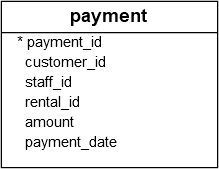### PostgreSQL `COUNT(*)` example

The following statement uses the `COUNT(*)` function to return the number of transactions in the `payment` table:

``````SELECT
COUNT(*)
FROM
payment;
``````

Here is the output: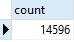### PostgreSQL `COUNT(DISTINCT column)` example

To get the distinct amounts which customers paid, you use the `COUNT(DISTINCT amount)` function as shown in the following example:

``````SELECT
COUNT (DISTINCT amount)
FROM
payment;
``````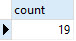### PostgreSQL `COUNT()` with `GROUP BY` clause

To get the number of payments by the customer, you use the `GROUP BY` clause to group the payments into groups based on customer id, and use the `COUNT()` function to count the payments for each group.

The following query illustrates the idea:

``````SELECT
customer_id,
COUNT (customer_id)
FROM
payment
GROUP BY
customer_id;
``````

Here is the partial output: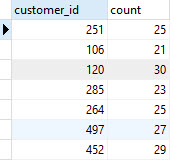### PostgreSQL `COUNT()` with `HAVING` clause

You can use the `COUNT` function in a `HAVING` clause to apply a specific condition to groups. For example, the following statement finds customers who have made more than 40 payments:

``````SELECT
customer_id,
COUNT (customer_id)
FROM
payment
GROUP BY
customer_id
HAVING
COUNT (customer_id) > 40;
``````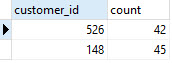In this tutorial, you have learned how to use the PostgreSQL `COUNT()` function to return the number of rows in a table.